Home Practice
For learners and parents For teachers and schools
Textbooks
Full catalogue
Pricing SupportLog in

We think you are located in United States. Is this correct?

# 2.4 Linear functions

## Worked example 4: Inverse of the function $$y=ax+q$$

Determine the inverse function of $$p(x) = -3x + 1$$ and sketch the graphs of $$p(x)$$ and $$p^{-1}(x)$$ on the same system of axes.

### Determine the inverse of the given function

• Interchange $$x$$ and $$y$$ in the equation.
• Make $$y$$ the subject of the new equation.
• Express the new equation in function notation.
\begin{align*} \text{Let } y & = -3x + 1 \\ \text{Interchange } x \text{ and } y: \quad x & = -3y + 1 \\ x - 1 & = -3y \\ -\frac{1}{3}(x - 1) & = y \\ \therefore y &= -\frac{x}{3} + \frac{1}{3} \end{align*}

Therefore, $$p^{-1}(x) = -\frac{x}{3} + \frac{1}{3}$$.

### Sketch the graphs of the same system of axes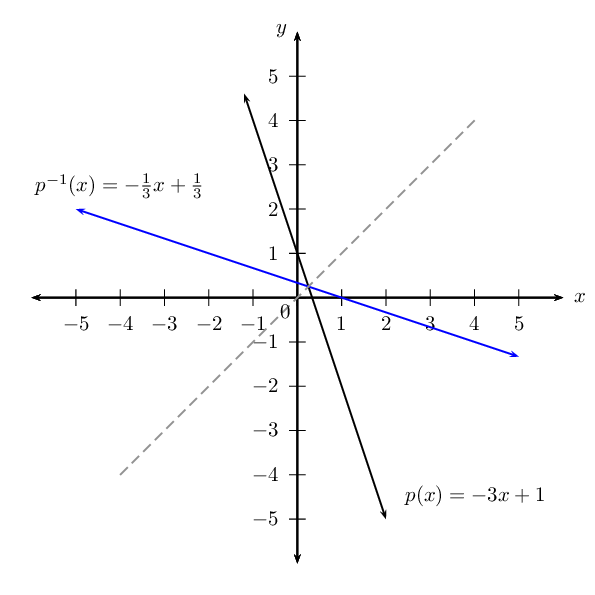The graph of $$p^{-1}(x)$$ is the reflection of $$p(x)$$ about the line $$y = x$$. This means that every point on the graph of $$p(x)$$ has a mirror image on the graph of $$p^{-1}(x)$$.

To determine the inverse function of $$y=ax+q$$:

$\begin{array}{rll} &(1) \quad \text{Interchange } x \text{ and } y: & x = ay+q \\ &(2) \quad \text{Make } y \text{ the subject of the equation}: & x - q = ay \\ && \frac{x}{a} - \frac{q}{a} = \frac{ay}{a} \\ &&\therefore y = \frac{1}{a}x-\frac{q}{a} \end{array}$

Therefore the inverse of $$y=ax+q$$ is $$y=\frac{1}{a}x-\frac{q}{a}$$. If a linear function is invertible, then its inverse will also be linear.

## Worked example 5: Inverses - domain, range and intercepts

Determine and sketch the inverse of the function $$f(x) = 2x - 3$$. State the domain, range and intercepts.

### Determine the inverse of the given function

• Interchange $$x$$ and $$y$$ in the equation.
• Make $$y$$ the subject of the new equation.
• Express the new equation in function notation.
\begin{align*} \text{Let } y & = 2x - 3 \\ \text{Interchange } x \text{ and } y: \quad x & = 2y - 3 \\ x + 3 & = 2y \\ \frac{1}{2}(x + 3) & = y \\ \therefore y &= \frac{x}{2} + \frac{3}{2} \end{align*}

Therefore, $$f^{-1}(x) = \frac{x}{2} + \frac{3}{2}$$.

### Sketch the graphs on the same system of axes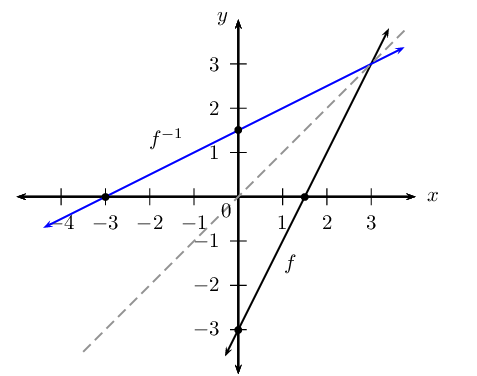The graph of $$f^{-1}(x)$$ is the reflection of $$f(x)$$ about the line $$y = x$$.

### Determine domain, range and intercepts

\begin{align*} \text{Domain of } f &: \{ x: x \in \mathbb{R} \} \\ \text{Range of } f &: \{ y: y \in \mathbb{R} \} \\ \text{Intercepts of } f &: (0;-3) \text{ and } \left(\frac{3}{2}; 0 \right) \\ & \\ \text{Domain of } f^{-1} &: \{ x: x \in \mathbb{R} \} \\ \text{Range of } f^{-1} &: \{ y: y \in \mathbb{R} \} \\ \text{Intercepts of } f^{-1} &: \left(0;\frac{3}{2}\right) \text{ and } (-3;0) \end{align*}

Notice that the intercepts of $$f$$ and $$f^{-1}$$ are mirror images of each other. In other words, the $$x$$- and $$y$$-values have “swapped” positions. This is true of every point on the two graphs.

#### Domain and range

For a function of the form $$y=ax+q$$, the domain is $$\left\{x:x\in ℝ\right\}$$ and the range is $$\left\{y:y\in ℝ\right\}$$. When a function is inverted the domain and range are interchanged. Therefore, the domain and range of the inverse of an invertible, linear function will be $$\left\{x:x\in ℝ\right\}$$ and $$\left\{y:y\in ℝ\right\}$$ respectively.

#### Intercepts

The general form of an invertible, linear function is $$y=ax+q \enspace (a \ne 0)$$ and its inverse is $$y=\frac{1}{a}x-\frac{q}{a}$$.

The $$y$$-intercept is obtained by letting $$x=0$$:

\begin{align*} y &= \frac{1}{a}(0)-\frac{q}{a} \\ y &= -\frac{q}{a} \end{align*}

This gives the point $$\left(0; -\frac{q}{a}\right)$$.

The $$x$$-intercept is obtained by letting $$y=0$$:

\begin{align*} 0 &= \frac{1}{a}x -\frac{q}{a} \\ \frac{q}{a} &= \frac{1}{a}x \\ q &= x \end{align*}

This gives the point $$(q; 0)$$.

It is interesting to note that if $$f\left(x\right)=ax+q \enspace (a \ne 0)$$, then $${f}^{-1}\left(x\right)=\frac{1}{a}x-\frac{q}{a}$$ and the $$y$$-intercept of $$f\left(x\right)$$ is the $$x$$-intercept of $${f}^{-1}\left(x\right)$$ and the $$x$$-intercept of $$f\left(x\right)$$ is the $$y$$-intercept of $${f}^{-1}\left(x\right)$$.

## Inverse of the function $$y = ax + q$$

Textbook Exercise 2.3

Given $$f\left(x\right)=5x + 4$$, find $${f}^{-1}\left(x\right)$$.

\begin{align*} f(x): y & = 5x + 4 \\ f^{-1}(x): x & = 5y + 4 \\ \therefore x - 4 & = 5y \\ y & = \frac{1}{5}x - \frac{4}{5}\\ \therefore f^{-1}(x) & = \frac{1}{5}x - \frac{4}{5} \end{align*}

Consider the relation $$f\left(x\right)=-3x-7$$.

It is a function. Every $$x$$-value relates to only one $$y$$-value, it is a one-to-one relation.

Identify the domain and range.

\begin{align*} \text{Domain } & \{ x: x \in \mathbb{R} \} \\ \text{Range } & \{ y: y \in \mathbb{R} \} \end{align*}

Determine $$f^{-1}(x)$$.

\begin{align*} f(x): y & = -3x-7 \\ f^{-1}(x): x & = -3y-7 \\ \therefore x + 7 & = -3y \\ y & = - \frac{1}{3}x - \frac{7}{3} \\ \therefore f^{-1}(x) & = - \frac{1}{3}x - \frac{7}{3} \end{align*}

Sketch the graph of the function $$f\left(x\right)=3x-1$$ and its inverse on the same system of axes. Indicate the intercepts and the axis of symmetry of the two graphs.

\begin{align*} y & = 3x - 1 \\ x & = 3y - 1\\ \therefore 3y & = x + 1 \\ y & = \frac{1}{3}x + \frac{1}{3} \end{align*}

The intercepts are:

\begin{align*} f(x): x = 0, y = -\text{1}\\ y=0, x = \frac{1}{3}\\ f^{-1}(x): x = 0, y = \frac{1}{3}\\ y = 0, x = -\text{1} \end{align*}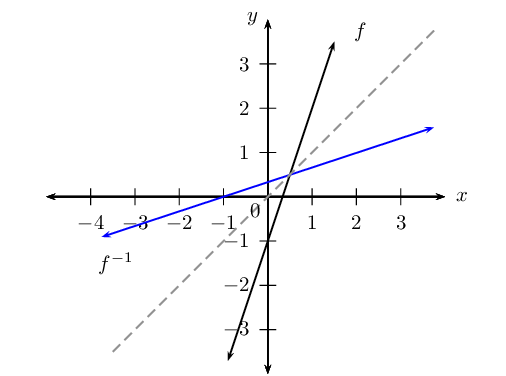$$T\left( \frac{4}{3}; 3 \right)$$ is a point on $$f$$ and $$R$$ is a point on $$f^{-1}$$. Determine the coordinates of $$R$$ if $$R$$ and $$T$$ are symmetrical.

$$R\left( 3; \frac{4}{3} \right)$$

Explain why the line $$y = x$$ is an axis of symmetry for a function and its inverse.

To reflect a function about the $$y$$-axis, we replace every $$x$$ with $$-x$$. Similarly, to reflect a function about the $$x$$-axis, we replace every $$y$$ with $$-y$$. To reflect a function about the line $$y=x$$, we replace $$x$$ with $$y$$ and $$y$$ with $$x$$, which is how we determine the inverse.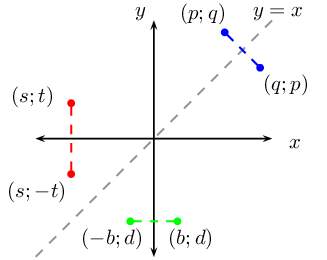Will the line $$y = -x$$ be an axis of symmetry for a function and its inverse?

No it will not.

Given $${f}^{-1}\left(x\right)=-2x+4$$, determine $$f\left(x\right)$$.

\begin{align*} f^{-1}(x): y & = -2x + 4 \\ f(x): x & = -2y + 4 \\ \therefore 2y & = -x + 4 \\ y & = -\frac{1}{2}x + 2\\ \therefore f(x) & = -\frac{1}{2}x + 2 \end{align*}

Calculate the intercepts of $$f(x)$$ and $$f^{-1}(x)$$.

Consider $$f(x)$$:

\begin{align*} \text{Let } y & = -\frac{1}{2}x + 2 \\ \text{Let } x=0: \quad y & = -\frac{1}{2}(0) + 2 \\ y & = 2 \\ \therefore y &-\text{intercept is } (0;2) \\ & \\ \text{Let } y=0: \quad 0 & = -\frac{1}{2}x + 2 \\ -2 & = -\frac{1}{2}x \\ x & = 4 \\ \therefore x &-\text{intercept is } (4;0) \end{align*}

Consider $$f^{-1}(x)$$:

\begin{align*} \text{Let } y & = - 2x+4 \\ \text{Let } x=0: \quad y & = - 2(0) + 4\\ y & = 4 \\ \therefore y &-\text{intercept is } (0;4) \\ & \\ \text{Let } y=0: \quad 0 & = - 2x+4\\ 2x & = 4 \\ x & = 2 \\ \therefore x &-\text{intercept is } (2;0) \end{align*}

Therefore the intercepts for $$f(x)$$ are $$(4;0)$$ and $$(0;2)$$ and the intercepts for $$f^{-1}(x)$$ are $$(2;0)$$ and $$(0;4)$$.

Determine the coordinates of $$T$$, the point of intersection of $$f(x)$$ and $$f^{-1}(x)$$.

To find the point on intersection, we let $$f(x) = f^{-1}(x)$$:

\begin{align*} -\frac{1}{2}x + 2 & = -2x + 4 \\ -\frac{1}{2}x + 2x & = 4 - 2 \\ \frac{3}{2}x & = 2 \\ \therefore x & = \frac{4}{3} \\ \text{And } y & = -2 \left( \frac{4}{3} \right) + 4 \\ & = - \frac{8}{3} + 4 \\ & = \frac{4}{3} \end{align*}

This gives the point $$T \left( \frac{4}{3};\frac{4}{3} \right)$$.

Sketch the graphs of $$f$$ and $$f^{-1}$$ on the same system of axes. Indicate the intercepts and point $$T$$ on the graph.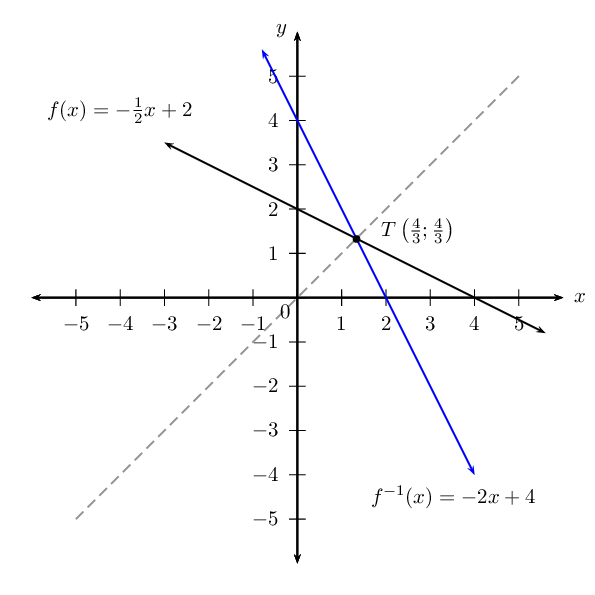Is $$f^{-1}$$ an increasing or decreasing function?

Decreasing function. The function values decrease as $$x$$ increases.